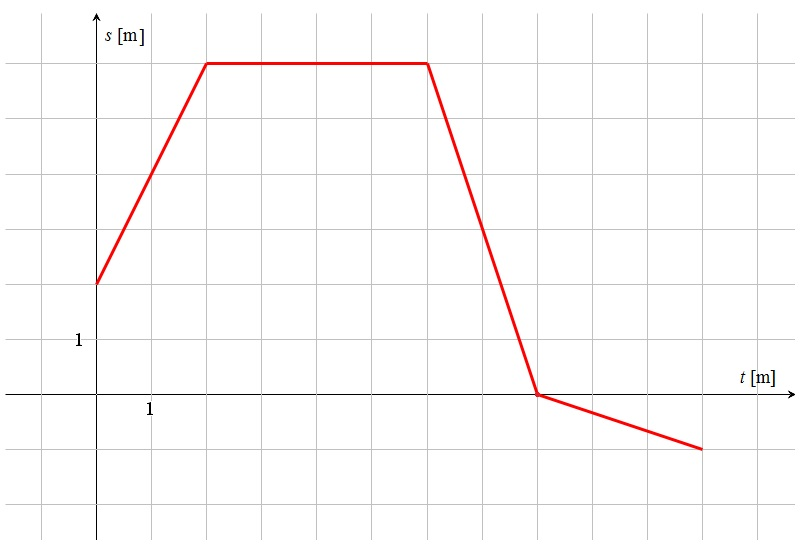# s/t-graphs

Up a level : Pre DP, Mechanics and Electronics
Previous page : More on velocity and speed
Next page : Quiz, s/t-graphss/t-graphs

Distance or displacement is often denoted by s (from Latin “spatium” for space)d (for distance or displacement),  h (for height)  or x, y and z (for coordinates).

A diagram describing how displacement (or distance) varies over time is called a s/t-graph or s/t-diagram.  Let us say the diagram below describes the movement of some object.The horizontal axis is labelled t [s] which means that it is indicating time and that the unit is seconds. This is also often written as t/m. The vertical axis is labelled with s [m], i.e. it is a displacement axis and the unit is metres.

At t=0 s we have s=2 m, This tells us that the object is  two meters away from a point we have defined as our origin in some direction we have defined as positive. Say the object us 2 meters to the east of a lamppost.

At t=2 s we have s=6 m.The object has thus moved four metres away from the previous point, and are now six metres away from the lamppost.

Since it has moved four metres in two seconds the average speed (and velocity since it is moving in the positive direction) is 4/2=2 m/s. This is also the slope of the straight line connecting the points (0, 2) and (2, 6).  This will be the velocity att all points through the fist two seconds since we have a straight line over these two seconds. More on that in the following page. We do in general have that:

• The velocity correspond to the slope (gradient) in an s/t-diagram.

It will then remain at six metres for four seconds. Then it will move back all the way to the lamppost in two seconds. The speed is thus 6/2=3 m/s. The velocity will be –3 m/s since the object is  moving back toward the lamppost.

One way to see this is that it moves –6 m in 3 s, and thus that the velocity is –6 m/s.

It then continues in the same direction, though somewhat slower. It will move –1 m in 3 s. The velocity is this –0.33 m/s and the speed is 0.33 m/s.

The total distance travelled will be 4+7=11 m (four meters forward + 7 meters backward) but the displacement will be –1 m since the object in the end is 1 meter away from the lamppost in the negative direction.

• The average speed is calculated  as the total distance divided by the total time taken.

In our example it would be 11 m / 11 s= 1 m/s for the whole motion.

In the interval from t=7 s to t=11 s we move from s=3 m to s= – 1 m. That would give us an average speed of$\bar v = \frac{{3 - ( - 1)}}{{11 - 7}} = \frac{4}{4} = 1{\rm{ m/s}}$

• The average velocity is calculated as the displacement divided by the total time.

In our example it would give us -1/11=0.91 m/s.

In the interval from t=7 s to t=11 s we move from s=3 m to s= – 1 m. That would give us an average velocity of$\bar v = \frac{{-1 - 3}}{{11 - 7}} = \frac{-4}{4} = -1{\rm{ m/s}}$Up a level : Pre DP, Mechanics and Electronics
Previous page : More on velocity and speed
Next page : Quiz, s/t-graphsLast modified: Jan 28, 2017 @ 10:19